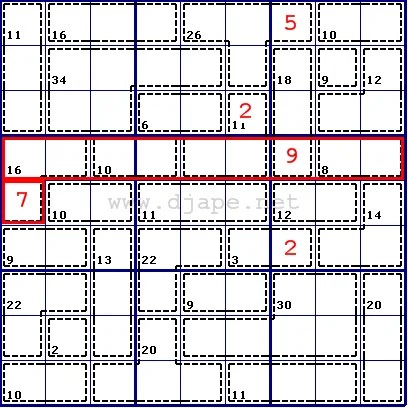# Cage Splitting – Killer Sudoku

Ok, here is the explanation of the “Cage/fence splitting” solving technique for Killer Sudoku puzzles that I promised before. I will use the puzzle posted on Friday, October 7 and solve it step by step using this method. We extend the idea of the “innies” and “outies” solving technique, except that you’re not looking for one cell but for any number of them. See the explanation of innies/outies. So, if you find, for instance, a row that has 7 cells that are in cages that completely belong to that row and the sum of all those cages is, say, 40, the remaining two cells must add up to 5, no matter in which column they are or if they’re next to one another or not. If that sounds too complicated, let’s go back to our sample puzzle. We’re focusing on the nonet number 6. It’s made of 3 cages plus 2 cells that belong to cages outside of it. The sum of those 3 cages is 8+12+14 = 34. Therefore, the other 2 cells must add up to (rule of 45) 45-34 = 11. Do you see the point now?Well, now you definitely do. One of those 2 cells belongs to a cage with sum of 3, which can only be 1+2. Since that cell must add up to 11 with another cell, 1 is simply not enough (you can’t have a 10 in the other cell). So it must be 2! Therefore, the last remaining cell in the nonet nr.6 must be 9.
If anyone is still with me, the rest should be pretty straightforward. Look at nonet 3. Cage marked with 18. We found that one of it’s cells must be 9. Therefore, we split that cage and figure out that it’s 2 cells belonging to nonet 3 must add up to 18-9 = 9. So we find an “innie” in the upper left corner of nonet 3: 45-(10+9+12+9) = 45 – 40 = 5.Next up – nonet nr.2. We split the cage marked with 26 because we know one of it’s cells (5), so it becomes 21. Then, we look at nonets 1 and 2 and find an “innie” of those two nonets put together. It’s value is 90-(11+16+34+6+21) = 90 – 88 = 2.The cell that we just solved (value 2) belongs to a cage marked with 11. We split that cage, so the part in the 5th nonet has the value 9. Now we look at the entire row nr.4. We see that almost all cages are completely within that row except for the cell at r5c1. So, it’s an “outie”! Rule of 45: (16+10+9+9+8) – 45 = 52 – 45 = 7. So, r5c1 = 7.From here, there are now many paths to the correct solution. In fact it can be solved by using only Sudoku techniques (as long as you make sure your cage-sums are Ok), but there are a few more cells that can be solved using innies/outies. The technique explained above can be quite complex depending on the puzzle. It’s quite possible that you will not be able to solve a cell by using it, but that you could remove some of the possible values for some cells. This often leads to another clue for some other cell. Hope this was helpful.

### One Comment

1.fiona
Posted December 3, 2011 at 3:38 am | Permalink

thanks for the help.I’m a newbie at killer. So much more absorbing and satisfying than sudoku.

Your explainations are very easy to take in and retain. I look forward to learning more from you site.

All the best

Fiona